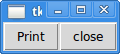# Python 101: Lambda Basics

Many programming languages have the concept of the lambda function. In Python, the lambda is an anonymous function or unbound function. The syntax for them looks a bit odd, but it's actually just taking a simple function and turning it into a one-liner. Let's look at a regular simple function to start off:

```#----------------------------------------------------------------------
def doubler(x):
return x*2
```

All this function does is take an integer and double it. Technically, it will also double other things too since there's no type checking but that is its intent Now let's turn it into a lambda function!

Fortunately, turning a one-line function into a lambda is pretty straight-forward. Here's how:

```doubler = lambda x: x*2
```

So the lambda works in much the same way as the function. Just so we're clear, the "x" here is the argument you pass in. The colon delineates the argument list from the return value or expression. So when you get to the "x*2" part, that is what gets returned. Let's look at another example:

```>>> poww = lambda i: i**2
>>> poww(2)
4
>>> poww(4)
16
>>> (lambda i: i**2)(6)
36
```

Here we demonstrate how to create a lambda that takes an input and squares it. There's some arguments in the Python community about whether or not you should assign a lambda to a variable. The reason is that when you name a lambda, it's no longer really anonymous and you might as well just write a regular function. The whole point of the lambda is to use it once and throw it away. The last example above shows one way to call a lambda anonymously (i.e. use it once).

Besides, if you name the lambda, then you might as well just do this:

```>>> def poww(i): return i**2
>>> poww(2)
4
```

Let's move on and see how to use a lambda in a list comprehension!

### Lambdas, List Comprehensions and map

I've always had a hard time coming up with good uses for lambdas besides using them for event callbacks. So I went looking for some other use cases. It seems that some people like to use them in list comprehensions. Here's a couple of examples:

```>>> [(lambda x: x*3)(i) for i in range(5)]
[0, 3, 6, 9, 12]
>>> tripler = lambda x: x*3
>>> [tripler(i) for i in range(5)]
[0, 3, 6, 9, 12]
```

The first example is a bit awkward. While it calls the lambda anonymously, it's also a bit difficult to read. The next example assigns the lambda to a variable and then we call it that way. The funny thing about this is that Python has a built-in way to call a function with an iterable called map. Here's how you would normally use it:

```map(function, interable)
```

And here's a real example using our lambda function from earlier:

```>>> map(tripler, range(5))
[0, 3, 6, 9, 12]
```

Of course, in most cases the lambda is so simple that doing the same thing inside the list comprehension is probably easier:

```>>> [x*3 for x in range(5)]
[0, 3, 6, 9, 12]
```

### Other uses of lambda

My readers have suggested some other uses of lambda. The first example is returning a lambda from a function call:

```>>> def increment(n):
return lambda(x): x + n
>>> i = increment(5)
>>> i(2)
7
```

Here we create a function that will increment whatever we give to it by 5. One of my other readers suggested passing a lambda to Python's sorted function:

```sorted(list, key=lambda i: i.address)
```

The idea here is to sort a list of objects with the property "address". However, I still find the best use case for lambda is still for event callbacks, so let's look at how to use them for that using Tkinter.

### Using lambda for CallbacksTkinter is a GUI toolkit that comes built-in with Python. When you want to interact with your user interface, you would normally use a keyboard and mouse. These interactions work via events, which is where the lambda makes its appearance. Let's create a simple user interface so you can see how lambda is used in Tkinter!

```import Tkinter as tk

########################################################################
class App:
""""""

#----------------------------------------------------------------------
def __init__(self, parent):
"""Constructor"""
frame = tk.Frame(parent)
frame.pack()

print_btn = tk.Button(frame, text='Print',
command=lambda: self.onPrint('Print'))
print_btn.pack(side=tk.LEFT)

close_btn = tk.Button(frame, text='close', command=frame.quit)
close_btn.pack(side=tk.LEFT)

#----------------------------------------------------------------------
def onPrint(self, num):
print "You just printed something"

#----------------------------------------------------------------------
if __name__ == "__main__":
root = tk.Tk()
app = App(root)
root.mainloop()
```

So here we have a simple user interface with two buttons. One button calls the onPrint method using a lambda while the other uses a Tkinter method to close the application. As you can see, Tkinter's use of the lambda is as it was intended. The lambda here is used once and then thrown away. There is no way to reference it other than by pressing the button.

### Wrapping Up

As my long time readers likely know, I wrote about the lambda in another article over five years ago. At that time, I didn't have much use for lambdas and frankly I still don't. They're a neat feature of the language, but after programming in Python for over 9 years, I've hardly ever found the need to use them, especially in the way that they are meant to be used. But to each their own. There's certainly nothing wrong with using lambdas, but I hope you'll find this article useful for figuring out whether or not you really do need to use them in your own work.